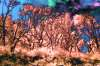Various Trees

article and photographs by Ben Lincoln

These trees were along one of the National Seashore beaches near Pensacola, Florida (see Drive 2010 - Day 6).

National Seashore 01R - G - BNIR - 87C Grey [Tinted]UVA - Neon Pink [Tinted]NIR - R - G [3C]NIR - G - B [3C]G - B - UVA [3C]All Bands (Max) - All Bands (Med) - All Bands (Min) [3C]All Bands (Skew) - All Bands (ADev) - All Bands (Range) [3C]Calculated: (Absolute Difference: NIR - G) - (Absolute Difference: NIR - B) - (Absolute Difference: NIR - UVA) [3C]Calculated: (Absolute Difference: R - UVA) - (Absolute Difference: G - UVA) - (Absolute Difference: B - UVA) [3C]Calculated: (Difference: NIR - UVA) - (Difference: R - UVA) - (Difference: B - UVA) [3C]Calculated: (Ratio: R To NIR) - (Ratio: B To NIR) - (Ratio: UVA To NIR) [3C]Custom: ClassicR72 [3C]Custom: PseudoR72 - Cyan [3C]Custom: PseudoR72 - Purple [3C]Custom: PseudoR72 - Yellow [3C](All Bands (ADev)) Luminance - (NIR - G - UVA) Colour [L/C](NIR) Luminance - (R - G - B) Colour [L/C](NIR) Luminance - (UVA - Tinted - 01) Colour [L/C]All Bands (ADev) Luminance - (Calculated: (Absolute Difference: R - G) - (Absolute Difference: G - B) - (Absolute Difference: B - UVA)) Colour [L/C]All Bands (ADev) Luminance - (Calculated: (ND (Pos): R - G) - (ND (Pos): R - B) - (ND (Pos): R - UVA)) Colour [L/C]All Bands (Var) Luminance - (Calculated: (ND (Pos): NIR - R) - (ND (Pos): R - G) - (ND (Pos): G - UVA)) Colour [L/C]NIR Luminance - (Calculated: (ND (Pos): R - G) - (ND (Pos): G - B) - (ND (Pos): B - UVA)) Colour [L/C]Experimental: DCS-Enhanced Contrast - (All Bands (Max) - All Bands (Med) - All Bands (Min)) - RDCS: (YUV - 25 Percent)Experimental: DCS-Enhanced Contrast - (R - G - B) - RDCS: (YUV - 25 Percent)Experimental: Gradient - Ratio Vegetation Index

I used this shot for testing various processing methods while I was building The Mirror's Surface Breaks, so there are a few unusual variations included.

Date Shot: 2010-07-10
Camera Body: Nikon D70 (Modified)
Lens: Nikkor-O 35mm f/2 @ f/?
Filters: Standard Set
Date Processed: 2011-05-07
Version: 1.1

This set of images is from an orange grove in Gardner, Florida (see Drive 2010 - Day 8).

Orange Grove (Gardner, Florida) 02R - G - BNIR - 87C Grey [Tinted]UVA - Neon Pink [Tinted]G - B - UVA [3C]All Bands (Max) - All Bands (Med) - All Bands (Min) [3C]Calculated: (Absolute Difference: NIR - R) - (Absolute Difference: R - G) - (Absolute Difference: G - UVA) [3C]Calculated: (Ratio: R To NIR) - (Ratio: G To NIR) - (Ratio: UVA To NIR) [3C]Calculated: (Ratio: R To NIR) - (Ratio: G To R) - (Ratio: UVA To G) [3C]Statistical: All Bands (ADev) - All Bands (SDev) - All Bands (Range) [3C]Statistical: All Bands (Kurt) - All Bands (Var) - All Bands (Range) [3C]Statistical: All Bands (Max) - All Bands (ADev) - All Bands (SDev) [3C]All Bands (ADev) Luminance - (Calculated: (ND (Pos): NIR - R) - (ND (Pos): NIR - B) - (ND (Pos): NIR - UVA)) Colour [L/C]All Bands (ADev) Luminance - (Calculated: (ND (Pos): NIR - R) - (ND (Pos): R - G) - (ND (Pos): G - UVA)) Colour [L/C]All Bands (ADev) Luminance - (Calculated: (ND (Pos): NIR - UVA) - (ND (Pos): R - UVA) - (ND (Pos): B - UVA)) Colour [L/C]All Bands (ADev) Luminance - (Calculated: (ND (Pos): R - G) - (ND (Pos): G - B) - (ND (Pos): B - UVA)) Colour [L/C]All Bands (ADev) Luminance - (Calculated: (Ratio: R To G) - (Ratio: G To B) - (Ratio: B To UVA)) Colour [L/C]All Bands (ADev) Luminance - (Calculated: (Ratio: UVA To NIR) - (Ratio: UVA To R) - (Ratio: UVA To B)) Colour [L/C]NIR Luminance - (Calculated: (ND (Pos): R - G) - (ND (Pos): R - B) - (ND (Pos): R - UVA)) Colour [L/C]NIR Luminance - (Calculated: (Ratio: R To NIR) - (Ratio: G To R) - (Ratio: B To G)) Colour [L/C]NIR Luminance - (Calculated: (Ratio: UVA To NIR) - (Ratio: UVA To R) - (Ratio: UVA To B)) Colour [L/C]NIR Luminance - (R - G - B) Colour [L/C]

Date Shot: 2010-07-12
Camera Body: Nikon D70 (Modified)
Lens: Nikkor-N 24mm f/2.8 @ f/?
Filters: Standard Set
Date Processed: 2011-05-07
Version: 1.1